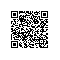# 致敬赵雷：基于TensorFlow让机器生成赵雷曲风的歌词【无法长大】共收录了10支单曲：

《朵》、《八十年代的歌》、《无法长大》、《玛丽》、《阿刁》、《鼓楼》、《孤独》、《成都》、《窑上路》、《再见北京》【吉姆餐厅】共收录了10支单曲：

《吉姆餐厅》、《少年锦时》、《梦中的哈德森》、《我们的时光》、《理想》、《三十岁的女人》、《家乡》、《浮游》、《小屋》、《北京的冬天》【赵小雷】共收录了12支单曲：

《人家》、《未给姐姐递出的信》、《画》、《不开的唇》、《赵小雷》、《南方姑娘》、《Over》、《开往北京的火车》、《背影》、《妈妈》、《南方姑娘（弹唱版）》、《民谣》【其他单曲】共22支单曲：

《19岁时候的歌》、《已是两条路上的人》、《再也不会去丽江》、《让我偷偷看你》、《咬春》、《难受》、《辞行》、《保存》、《雪人》、《青春无处安放》、《凭什么说爱你》、《过年》、《何必》、《2012年之前》、《别》、《爱人你在哪里》、《夏天》、《飞来飞去》、《逆流而上》、《花朵》、《罪》、《不能自主》

1.原理回顾• 序列A中的每一个单词通过word_embedding操作以后，作为input进入编码器，编码器可以是一个多层RNN结构，编码器输出一个向量；

• 训练的时候，解码器的输入跟编码器的输入是一样的，然后解码器的输出与序列B之间的交叉熵作为模型的目标函数；

• 生成的时候，首先给定一个种子序列作为编码器的输入，并且解码器的上一时刻的输出作为下一时刻的输入，如此循环往复，直到生成给定数量的序列。

2.模型代码设计• 主目录下面的utils.py是公共函数库，preprocess.py是数据预处理代码，seq2seq_rnn.py是模型代码，sample.py是抽样生成过程，train.py是训练过程；

• log目录中存储的是训练过程中的日志文件；

• save目录中存储的是训练过程中的模型存储文件；

• data目录中存放的是原始歌词数据库以及处理过的数据库；

• result目录中存放的是生成的序列；

• analysis目录中存放的是用于可视化的代码文件。

3.数据预处理

reg = re.compile(ur"[\s+]")

c = reg.sub(' ',unicode(c))

reg = re.compile(ur"[^\u4e00-\u9fa5\s]")

c = reg.sub('',unicode(c))

c = c.strip()

def build_dataset(self):
''' parse all sentences to build a vocabulary

dictionary and vocabulary list

'''
with codecs.open(self.input_file, "r",encoding='utf-8') as f:
wordCounts = collections.Counter(data)
self.vocab_list = [x for x in wordCounts.most_common()]
self.vocab_size = len(self.vocab_list)
self.vocab_dict = {x: i for i, x in enumerate(self.vocab_list)}
with codecs.open(self.vocab_file, 'wb',encoding='utf-8') as f:
cPickle.dump(self.vocab_list, f)
self.context = np.array(list(map(self.vocab_dict.get, data)))
np.save(self.context_file, self.context)

def init_batches(self):
'''

Split the dataset into mini-batches,

xdata and ydata should be the same length here

we add a space before the context to make sense.

'''

self.num_batches = int(self.context.size / (self.batch_size * self.seq_length))
self.context = self.context[:self.num_batches * self.batch_size * self.seq_length]
xdata = self.context
ydata = np.copy(self.context)
ydata[:-1] = xdata[1:]
ydata[-1] = xdata
self.x_batches = np.split(xdata.reshape(self.batch_size, -1), self.num_batches, 1)
self.y_batches = np.split(ydata.reshape(self.batch_size, -1), self.num_batches, 1)
self.pointer = 0

def next_batch(self):
''' pointer for outputing mini-batches when training

'''
x, y = self.x_batches[self.pointer], self.y_batches[self.pointer]
self.pointer += 1
if self.pointer == self.num_batches:
self.pointer = 0
return x, y

4.编写基于LSTM的seq2seq模型

• 确定好编码器和解码器中cell的结构，即采用什么循环单元，多少个神经元以及多少个循环层；

• 将输入数据转化成tensorflow的seq2seq.rnn_decoder需要的格式，并得到最终的输出以及最后一个隐含状态；

• 将输出数据经过softmax层得到概率分布，并且得到误差函数，确定梯度下降优化器。

if args.rnncell == 'rnn':
cell_fn = rnn_cell.BasicRNNCellelif args.rnncell == 'gru':
cell_fn = rnn_cell.GRUCellelif args.rnncell == 'lstm':
cell_fn = rnn_cell.BasicLSTMCellelse:
raise Exception("rnncell type error: {}".format(args.rnncell))

cell = cell_fn(args.rnn_size)

self.cell = rnn_cell.MultiRNNCell([cell] * args.num_layers)

decoder_inputs: A list of 2D Tensors [batch_size x input_size].
initial_state: 2D Tensor with shape [batch_size x cell.state_size].
cell: rnn_cell.RNNCell defining the cell function and size.
loop_function: If not None, this function will be applied to the i-th output
in order to generate the i+1-st input, and decoder_inputs will be ignored,
except for the first element ("GO" symbol). This can be used for decoding,
but also for training to emulate [1506.03099] Scheduled Sampling for Sequence Prediction with Recurrent Neural Networks.
Signature -- loop_function(prev, i) = next
* prev is a 2D Tensor of shape [batch_size x output_size],
* i is an integer, the step number (when advanced control is needed),
* next is a 2D Tensor of shape [batch_size x input_size].

self.input_data = tf.placeholder(tf.int32, [args.batch_size, args.seq_length])with tf.variable_scope('rnnlm'):

softmax_w = build_weight([args.rnn_size, args.vocab_size],name='soft_w')

softmax_b = build_weight([args.vocab_size],name='soft_b')

word_embedding = build_weight([args.vocab_size, args.embedding_size],name='word_embedding')

inputs_list = tf.split(1, args.seq_length, tf.nn.embedding_lookup(word_embedding, self.input_data))

inputs_list = [tf.squeeze(input_, ) for input_ in inputs_list]

initial_state是cell的初始状态，其维度是[batch_size，cell.state_size]，由于rnn_cell模块提供了对状态的初始化函数，因此我们可以直接调用：

self.initial_state = self.cell.zero_state(args.batch_size, tf.float32)

cell就是我们要构建的解码器和编码器的cell，上面已经提过了。最后一个参数是loop_function，其作用是在生成的时候，我们需要把解码器上一时刻的输出作为下一时刻的输入，并且这个loop_function需要我们自己写，如下所示：

def loop(prev, _):
prev = tf.matmul(prev, softmax_w) + softmax_b
return tf.nn.embedding_lookup(embedding, prev_symbol)

outputs, last_state = seq2seq.rnn_decoder(inputs_list, self.initial_state, self.cell, loop_function=loop if infer else None, scope='rnnlm')

tensorflow中提供了sequence_loss_by_example函数用于按照权重来计算整个序列中每个单词的交叉熵，返回的是每个序列的log-perplexity。为了使用sequence_loss_by_example函数，我们首先需要将outputs通过一个前向层，同时我们需要得到一个softmax概率分布，这个在生成中会用到：

output = tf.reshape(tf.concat(1, outputs), [-1, args.rnn_size])

self.logits = tf.matmul(output, softmax_w) + softmax_b

self.probs = tf.nn.softmax(self.logits)

loss = seq2seq.sequence_loss_by_example([self.logits],
[tf.reshape(self.targets, [-1])],
[tf.ones([args.batch_size * args.seq_length])],
args.vocab_size)

# average loss for each word of each timestepself.cost = tf.reduce_sum(loss) / args.batch_size / args.seq_length

self.lr = tf.Variable(0.0, trainable=False)

self.var_trainable_op = tf.trainable_variables()

self.initial_op = tf.initialize_all_variables()

self.saver = tf.train.Saver(tf.all_variables(),max_to_keep=5,keep_checkpoint_every_n_hours=1)

self.logfile = args.log_dir+str(datetime.datetime.strftime(datetime.datetime.now(),'%Y-%m-%d %H:%M:%S')+'.txt').replace(' ','').replace('/','')

self.var_op = tf.all_variables()

train_op即为训练时需要运行的。

5.编写抽样生成函数

state = sess.run(self.cell.zero_state(1, tf.float32))

for word in start:
x = np.zeros((1, 1))
x[0, 0] = words[word]
feed = {self.input_data: x, self.initial_state:state}
[probs, state] = sess.run([self.probs, self.final_state], feed)

• argmax型：即找出probs中最大值所对应的索引，然后去单词表中找到该索引对应的单词即为最佳单词；

• weighted型：即随机取样，其工作流程如下：首先，计算此probs的累加总和S；其次，随机生成一个0~1之间的随机数，并将其与probs的总和相乘得到R；最后，将R依次减去probs中每个数，直到R变成负数的那个probs的索引，即为我们要挑选的最佳单词；

• combined型： 这里我把argmax和weighted结合起来了，即每次遇到一个空格（相当于一句歌词的结尾），就使用weighted型，而其他时候都使用argmax型。

def random_pick(p,word,sampling_type):
def weighted_pick(weights):
t = np.cumsum(weights)
s = np.sum(weights)
return(int(np.searchsorted(t, np.random.rand(1)*s)))
if sampling_type == 'argmax':
sample = np.argmax(p)
elif sampling_type == 'weighted':
sample = weighted_pick(p)
elif sampling_type == 'combined':
if word == ' ':
sample = weighted_pick(p)
else:
sample = np.argmax(p)
return sample

word = start[-1]for n in range(num):
x = np.zeros((1, 1))
x[0, 0] = words[word]
if not self.args.attention:
feed = {self.input_data: [x], self.initial_state:state}
[probs, state] = sess.run([self.probs, self.final_state], feed)
else:
feed = {self.input_data: x, self.initial_state:state,self.attention_states:attention_states}
[probs, state] = sess.run([self.probs, self.final_state], feed)
p = probs
sample = random_pick(p,word,sampling_type)
pred = vocab[sample]
ret += pred
word = pred

ret数组即为最终的生成序列。

6.编写训练函数

help='set size of RNN hidden state')

python train.py --rnn_size 256

if args.keep is True:
print('Restoring')
model.saver.restore(sess, ckpt.model_checkpoint_path)

else:
print('Initializing')
sess.run(model.initial_op)

x, y = text_parser.next_batch()

feed = {model.input_data: x, model.targets: y, model.initial_state: state}

train_loss, state, _ = sess.run([model.cost, model.final_state, model.train_op], feed)

7.编写日志

if (e*text_parser.num_batches+b)%args.save_every==0 、
or (e==args.num_epochs-1 and b==text_parser.num_batches-1):
checkpoint_path = os.path.join(args.save_dir, 'model.ckpt')
model.saver.save(sess, checkpoint_path, global_step = e)
print("model has been saved in:"+str(checkpoint_path))

delta_time = end - start

ave_loss = np.array(total_loss).mean()

logging(model,ave_loss,e,delta_time,mode='train')

8.编写可视化函数

if line.startswith('Epoch'):
if 'validate' in line:
index2 = index2 + 1
cost = line.split(':')
indexValidateList.append(index2)
validateCostList.append(float(cost))
elif 'train error rate' in line:
index1 = index1+1
cost = line.split(':')
indexCostList.append(index1)
costList.append(float(cost))

def plot(self):
title,indexCostList,costList = self.parse()
p1 = plt.plot(indexCostList,costList,marker='o',color='b',label='train cost')
plt.xlabel('Epoch')
plt.ylabel('Cost')
plt.legend()
plt.title(title)
if self.saveFig:
plt.savefig(self.logFile+'.png',dpi=100)
#plt.savefig(self.logFile+'.eps',dpi=100)
if self.showFig:
plt.show()

9.设置训练超参

10.训练环境

11.结果展示

12.优化改进

“平淡” ： 赵雷为人就是怎么平易近人

“有你们的爱”： 给所有爱赵雷的人们

“游戏而已”： 赵雷在歌手说过的一句话

“我就是我”： 赵雷你是不一样的烟火

“北方姑娘”： 赵雷南方姑娘的对立

“我的姑娘你在哪儿”： 希望赵雷找到自己的幸福